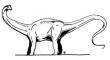Search IntMath
Close

450+ Math Lessons written by Math Professors and Teachers

5 Million+ Students Helped Each Year

1200+ Articles Written by Math Educators and Enthusiasts

Simplifying and Teaching Math for Over 23 Years

# 1. Polynomial Functions and Equations

## What is a Polynomial?

Before we look at the formal definition of a polynomial, let's have a look at some graphical examples.

In this interactive graph, you can see examples of polynomials with degree ranging from 1 to 8. The degree of a polynomial is the highest power of x that appears.

The "a" values that appear below the polynomial expression in each example are the coefficients (the numbers in front of) the powers of x in the expression.

The pink dots indicate where each curve intersects the x-axis. These are called the roots (or zeros) of the polynomial equation f(x) = 0.

### Things to do

1. Use the "Degree" + and − buttons below the graph to change the degree of the polynomial.
2. Use the "an slider" below the graph to move the graph up and down.
3. Observe that:
• A degree 1 polynomial has at most 1 root
• A degree 2 polynomial has at most 2 roots
• A degree 3 polynomial has at most 3 roots
• ... etc.
Degree =
a0

#### Polynomial of degree

Note 1: These are "typical" shapes for such polynomials. It's also possible they can be stretched out such that they have less roots.

Note 2: Of course, we are restricting ourselves to real roots for the moment.

## Formal definition of a polynomial

A polynomial function of degree n is of the form:

f(x) = a0xn + a1xn−1 + a2xn−2 + ... + an

where

a0 ≠ 0 and

n is a positive integer, called the degree of the polynomial.

### Example

f(x) = x4 x3 − 19x2 − 11x + 31 is a polynomial function of degree 4.

## Roots of an Equation

Finding the roots of a polynomial equation, for example

x4 x3 − 19x2 − 11x + 31 = 0,

means "to find values of x which make the equation true."

We'll find those roots using a computer algebra system instead of using the (quite useless) Factor and Remainder Theorems. (I have reluctantly included them in the next section, Remainder and Factor Theorems).

This is because the Factor and Remainder Theorems only really work for "nice" polynomials (that is, ones which have integer coefficients and so are easy to guess an initial root).

It's usually best to draw a graph of the function and determine the roots from where the graph cuts the x-axis. Let's see how that works.

Solution 1: Graphically. The roots are given by the x-intercepts.

f(x) = x4 x3 − 19x2 − 11x + 31

Graph of f(x) = x4 x3 − 19x2 − 11x + 31

We see that there are 4 roots, at approximately

x = -3, x = -2, x = 1, x = 5.

Solution 2: Using "Compute → Solve → Numeric" in Scientific Notebook (or similar processes in other computer algebra systems):

f(x) = x4 − x3 − 19x2 − 11x + 31,

Solution is: {x = -2.97}, {x = -2.05}, {x = 1.02}, {x = 4.99}.

We see that the roots are close to our estimation from the graph.So you still insist on seeing the dinosaur method of solving these? Okay, here you go...

In the next section we meet the Remainder Theorem and Factor Theorem, which were historically used to find solutions of polynomial equations. They are only useful for polynomial equations with simple roots.

## Problem SolverThis tool combines the power of mathematical computation engine that excels at solving mathematical formulas with the power of GPT large language models to parse and generate natural language. This creates math problem solver thats more accurate than ChatGPT, more flexible than a calculator, and faster answers than a human tutor. Learn More.